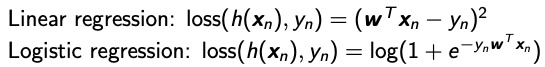# Request to confirm Formula for Log Regression loss

Folks - Pretty new to PyTorch . I am trying to write a logistic regression loss using PyTorch.
Math Formula:## Is the below implementation for the second math formula for Log Reg Correct ?

``````class Regress_Loss(nn.modules.Module):
def __init__(self):
super(Regress_Loss,self).__init__()

def forward(self, outputs, labels):
batch_size = outputs.size()